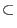Next: Quantum Algorithms Up: The Quantum Computer Previous: Quantum Parallelism   Contents

## The Efficiency of the Quantum Computer

Given the possible efficiency of quantum parallelism much work has been done to show formally with mathematical proofs how quantum computers differ from classical ones in the complexity classes and running times of various algorithms. Here is a short list of some of the landmarks in the study of the efficiency of quantum computers.

In 1980 Paul Benioff offered a classical Turing machine which used quantum mechanics in its workings, thus showing that theoretically a quantum computer was at least as powerful as a classical computer. (Benioff)

In 1982 Richard Feynman showed that a classical Turing Machine (and hence any classical computer if the Complexity-Theoretic Church-Turing Thesis holds) could not simulate a quantum mechanical system without suffering exponential slowdown. (Feynman)

David Deutsch and Richard Jozsa showed in a paper in 1992 that there was an algorithm that could be run in poly-log time on a quantum computer, but required linear time on a deterministic Turing machine. This may have been the first example of a quantum computer being shown to be exponentially faster than a deterministic Turing machine. However, the problem could also be solved in poly-log time in a probabilistic Turing machine, a Turing machine which is capable of making a random choice. (Deutsch, Jozsa)

Andre Berthiaume and Giles Brassard proved in a 1992 paper that PQP, where P is a complexity class as mentioned earlier and QP (also denoted as EQP) corresponds to problems which can be solved in worst case polynomial time by a quantum computer, so there are indeed problems which can be solved in polynomial time on a quantum computer that can not be solved in a polynomial time with a deterministic Turing machine. (Berthiaume, Brassard)Next: Quantum Algorithms Up: The Quantum Computer Previous: Quantum Parallelism   Contents
Matthew Hayward - Quantum Computing and Shor's Algorithm GitHub Repository